# CHAID Decision Trees (R)

## Decision Trees¶

Decision trees are a collection of predictive analytic techniques that use tree-like graphs for predicting the response variable. One such method is CHAID. Decision trees partition the data set into mutually exclusive and exhaustive subsets, which results in the splitting of the original data resembling a tree-like structure.

## CHAID¶

We can use Chi-square automatic interaction detection for classifying categorical variables when we have only categorical predictors. In CHAID, we categorise the data based on the following hypothesis tests:
1. Chi-square Test of Independence when the response variable, Y, is discrete
2. F-test when the response variable, Y, is continuous
3. Likelihood Ratio Test when the response variable, Y, is ordinal

The steps involved in developing a CHAID tree are
2. Check the statistical significance of each independent variable depending on the type of dependent variable
3. The variable with the least p-value, based on the statistical tests is used for splitting the dataset, thereby creating subsets. (We can use Bonferroni correction for adjusting the significance level alpha. We can merge the non-significant categories in a categorical predictor variable with more than two groups)
4. Using independent variables, repeat step 3 for each of the subsets of the data until
(a) All the dependent variables are exhausted, or they are not statistically significant at alpha
(b) We meet the stopping criteria
5. Generate business rules for the terminal nodes (nodes without any branches) of the tree

The data used in this blog is the same as the used in other classification posts, i.e. the Titanic dataset from Kaggle. In this problem, we have to identify who has a higher chance of survival.

titanic dataset
Survived Pclass Sex Age SibSp Parch Fare Embarked
O 3 male 21.00 0 0 16.1000 S
O 1 male 38.00 0 1 153.4625 S
O 1 male 28.00 0 0 47.1000 S
I 1 female 42.00 0 0 227.5250 C
O 3 male 27.85 0 0 7.8958 S

### Step 2: Statistical significance of each variable¶

In this dataset, Pclass, Gender, SibSp, Parch, Embarked are taken as categorical variables. For categorical variables, the Chi-square Test of Independence test is performed with the null hypothesis ($H_0$) the independent variable and Survival are independent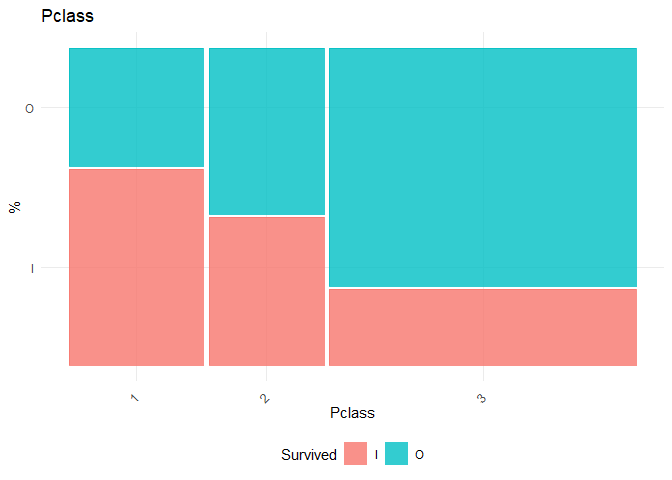##  "Chi-square test for Pclass"
##
##  Pearson's Chi-squared test
##
## data:  as.factor(list_x) and as.factor(list_y)
## X-squared = 100.98, df = 2, p-value < 2.2e-16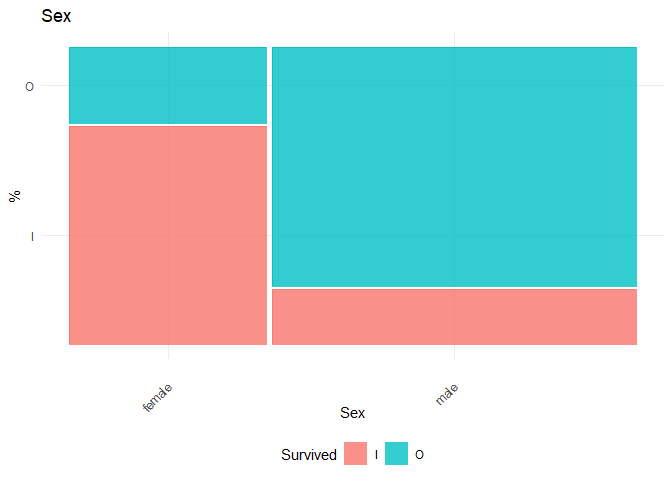##  "Chi-square test for Sex"
##
##  Pearson's Chi-squared test with Yates' continuity correction
##
## data:  as.factor(list_x) and as.factor(list_y)
## X-squared = 258.43, df = 1, p-value < 2.2e-16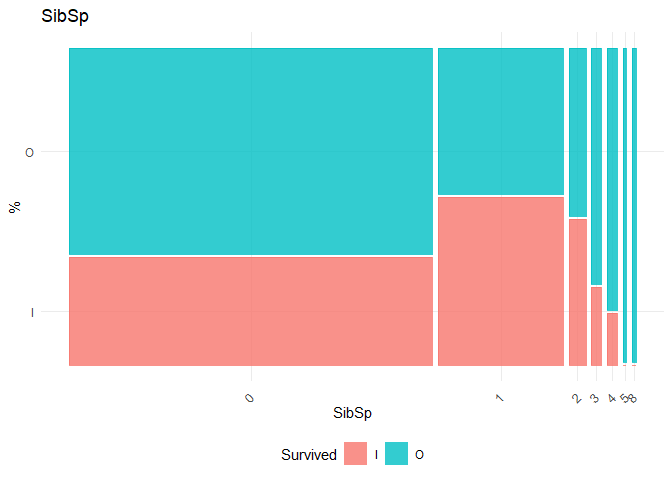##  "Chi-square test for SibSp"
##
##  Pearson's Chi-squared test
##
## data:  as.factor(list_x) and as.factor(list_y)
## X-squared = 37.741, df = 6, p-value = 1.262e-06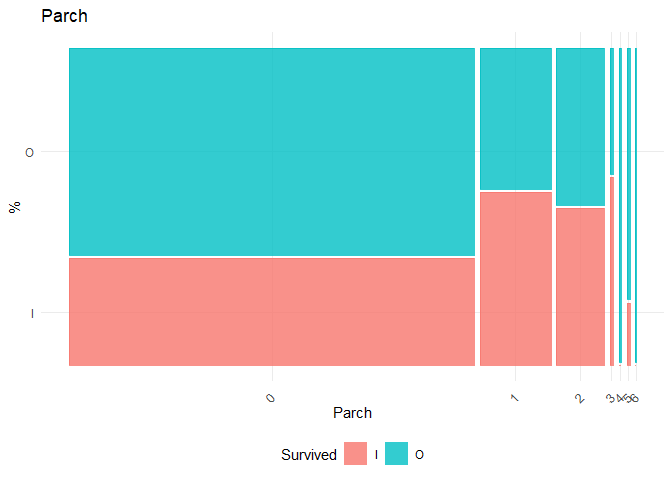##  "Chi-square test for Parch"
##
##  Pearson's Chi-squared test
##
## data:  as.factor(list_x) and as.factor(list_y)
## X-squared = 28.401, df = 6, p-value = 7.896e-05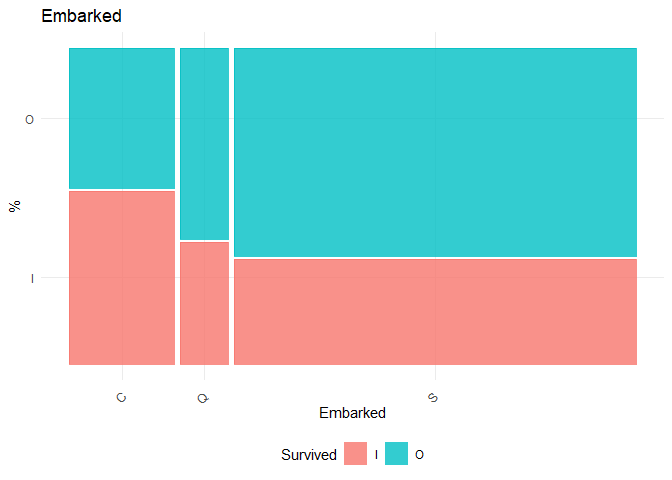##  "Chi-square test for Embarked"
##
##  Pearson's Chi-squared test
##
## data:  as.factor(list_x) and as.factor(list_y)
## X-squared = 26.489, df = 2, p-value = 1.77e-06


Age and Fare are the continuous variables. For continuous variables, ANOVA is performed with the null hypothesis $$H_0: \mu_{class 1} = ...=\mu_{class n}$$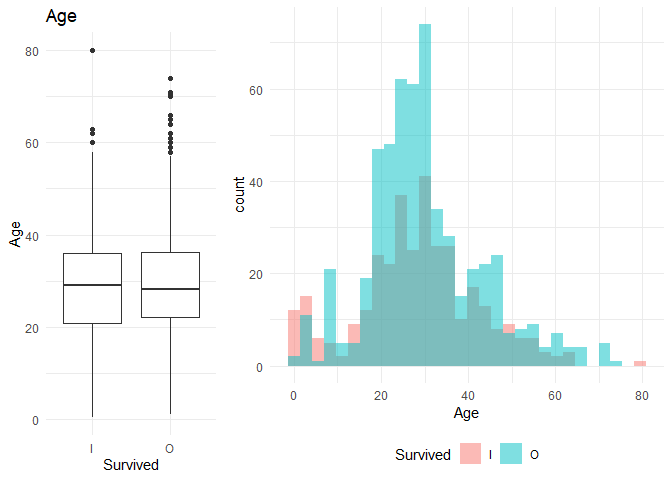##  "Age"
##              Df Sum Sq Mean Sq F value Pr(>F)
## Survived      1    531   530.8   2.917  0.088 .
## Residuals   887 161371   181.9
## ---
## Signif. codes:  0 '***' 0.001 '**' 0.01 '*' 0.05 '.' 0.1 ' ' 1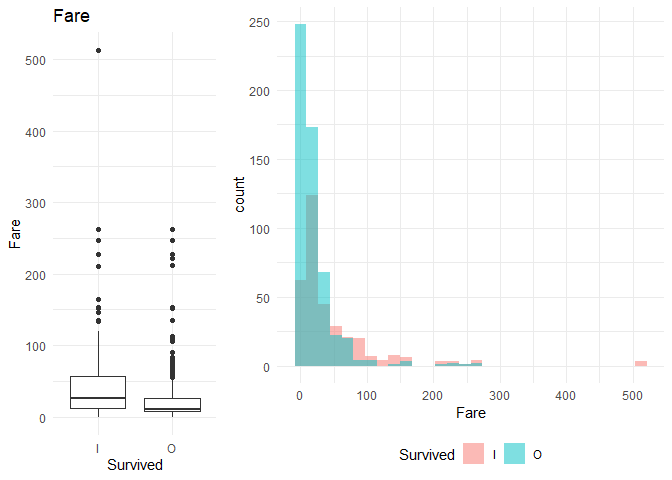##  "Fare"
##              Df  Sum Sq Mean Sq F value   Pr(>F)
## Survived      1  142939  142939   61.84 1.08e-14 ***
## Residuals   887 2050280    2311
## ---
## Signif. codes:  0 '***' 0.001 '**' 0.01 '*' 0.05 '.' 0.1 ' ' 1


### Step 3: Selecting the best variable to split based on least p-value¶

For all the dependant variables, the summary of the tests and the test statistic, along with the p-value, is given below:

CHAID
predictor test df statistic probability
Pclass chi-Sq 2 100.980407 0.0000000
Sex chi-Sq 1 258.426610 0.0000000
SibSp chi-Sq 6 37.741349 0.0000013
Parch chi-Sq 6 28.400619 0.0000790
Embarked chi-Sq 2 26.489150 0.0000018
Age F test 1887, 2.917453 0.0879760
Fare F test 1887, 61.838885 0.0000000

As the p-value for gender is the least, the first split takes place based on gender. Therefore the first split is done based on gender.

##
##     female  male
##   I  74.04 34.94
##   O  14.04 81.11


### Step 4: Repeting steps 1,2 and 3 until the stopping criterion¶

We repeat steps 1, 2 and 3 unless the minimum data points in a leaf are at least 100(stopping criterion) or till the probability value is less than 5 per cent. The final tree is as follows: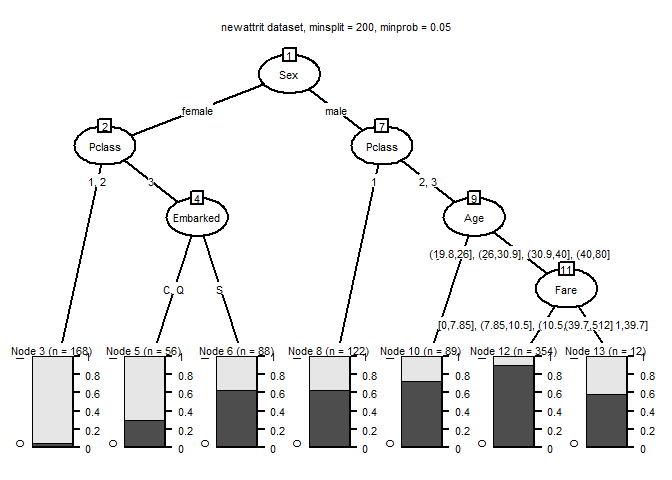The business rules for the tree can be obtained as:

##
## Model formula:
## Survived ~ Pclass + Sex + Age + SibSp + Parch + Fare + Embarked
##
## Fitted party:
##  root
## |    Sex in female
## |   |    Pclass in 1, 2: I (n = 168, err = 5.4%)
## |   |    Pclass in 3
## |   |   |    Embarked in C, Q: I (n = 56, err = 30.4%)
## |   |   |    Embarked in S: O (n = 88, err = 37.5%)
## |    Sex in male
## |   |    Pclass in 1: O (n = 122, err = 36.9%)
## |   |    Pclass in 2, 3
## |   |   |    Age in [0.42,19.8]: O (n = 89, err = 27.0%)
## |   |   |    Age in (19.8,26], (26,30.9], (30.9,40], (40,80]
## |   |   |   |    Fare in [0,7.85], (7.85,10.5], (10.5,21.1], (21.1,39.7]: O (n = 354, err = 9.9%)
## |   |   |   |    Fare in (39.7,512]: O (n = 12, err = 41.7%)
##
## Number of inner nodes:    6
## Number of terminal nodes: 7